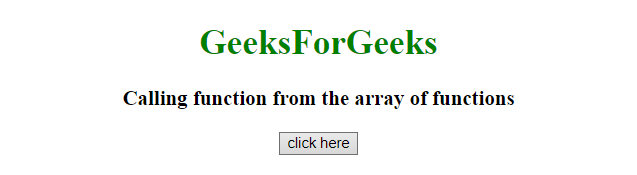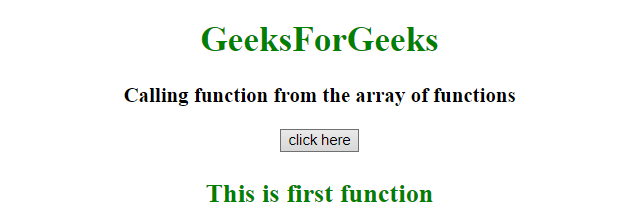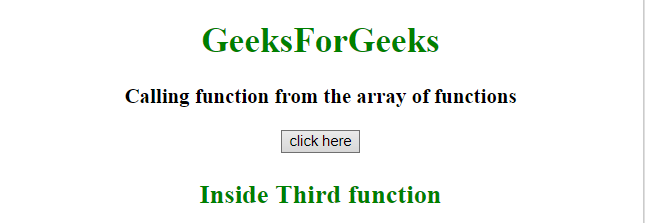# Array of functions in JavaScript

Given an array containing functions and the task is to access its element in different ways using JavaScript.

Approach:

• Declare an array of functions.
• The array of function works with indexes like an array function.

Example 1: In this example, the function call is initiated from the element of array but the function is defined somewhere else. We can pass arguments to the function while calling.

 `  ` `<``html``>  ` `    ``<``head``>  ` `        ``<``title``>  ` `            ``Array of functions in javascript ` `        `` ` `    ``  ` `     `  `    ``<``body` `style` `= ``"text-align:center;"``>  ` `         `  `        ``<``h1` `style` `= ``"color:green;"` `>  ` `            ``GeeksForGeeks  ` `        `` ` `         `  `        ``<``p` `id` `= ``"GFG_UP"` `style` `= ` `            ``"font-size: 19px; font-weight: bold;"``> ` `        `` ` `         `  `        ``<``button` `onClick` `= ``"GFG_Fun()"``> ` `            ``click here ` `        `` ` `         `  `        ``<``p` `id` `= ``"GFG_DOWN"` `style` `=  ` `            ``"color: green; font-size: 24px; font-weight: bold;"``> ` `        `` ` `         `  `        ``<``script``> ` `            ``var up = document.getElementById('GFG_UP'); ` `            ``var down = document.getElementById('GFG_DOWN'); ` `             `  `            ``function firstFun(str) { ` `                ``down.innerHTML = str; ` `            ``} ` `            ``function secondFun(str) { ` `                ``down.innerHTML = str; ` `            ``} ` `            ``function thirdFun(str) { ` `                ``down.innerHTML = str; ` `            ``} ` `             `  `            ``// Declare array of functions ` `            ``var arrayOfFunction = [ ` `                ``firstFun, ` `                ``secondFun, ` `                ``thirdFun      ` `            ``] ` `             `  `            ``up.innerHTML = "Calling function from the array of functions"; ` `             `  `            ``// Function call ` `            ``function GFG_Fun() { ` `                ``arrayOfFunction("This is first function"); ` `            ``} ` `        ``  ` `    ``  ` `                     `

Output:

• Before clicking on the button:• After clicking on the button:Example 2: In this example, the function (anonymous) itself is defined as the elements of array. We can access it by accessing the element of array followed by ().

 `  ` `<``html``>  ` `    ``<``head``>  ` `        ``<``title``>  ` `            ``Array of functions in JavaScript ` `        `` ` `    ``  ` `     `  `    ``<``body` `style` `= ``"text-align:center;"``>  ` `         `  `        ``<``h1` `style` `= ``"color:green;"` `>  ` `            ``GeeksForGeeks  ` `        `` ` `         `  `        ``<``p` `id` `= ``"GFG_UP"` `style` `=  ` `            ``"font-size: 19px; font-weight: bold;"``> ` `        `` ` `         `  `        ``<``button` `onClick` `= ``"GFG_Fun()"``> ` `            ``click here ` `        `` ` `         `  `        ``<``p` `id` `= ``"GFG_DOWN"` `style` `= ` `            ``"color: green; font-size: 24px; font-weight: bold;"``> ` `        `` ` `         `  `        ``<``script``> ` `            ``var up = document.getElementById('GFG_UP'); ` `            ``var down = document.getElementById('GFG_DOWN'); ` `             `  `            ``// Declare an array of functions ` `            ``var arrayOfFunction = [ ` `                ``function() { ` `                    ``down.innerHTML = "Inside First function"; ` `                ``}, ` `                 `  `                ``function() { ` `                    ``down.innerHTML = "Inside Second function"; ` `                ``}, ` `                 `  `                ``function() { ` `                    ``down.innerHTML = "Inside Third function"; ` `                ``}      ` `            ``] ` `             `  `            ``up.innerHTML = "Calling function from the array of functions"; ` `             `  `            ``function GFG_Fun() { ` `                ``arrayOfFunction(); ` `            ``} ` `        ``  ` `    ``  ` `                     `

Output:

• Before clicking on the button:• After clicking on the button:My Personal Notes arrow_drop_upCheck out this Author's contributed articles.

If you like GeeksforGeeks and would like to contribute, you can also write an article using contribute.geeksforgeeks.org or mail your article to contribute@geeksforgeeks.org. See your article appearing on the GeeksforGeeks main page and help other Geeks.

Please Improve this article if you find anything incorrect by clicking on the "Improve Article" button below.